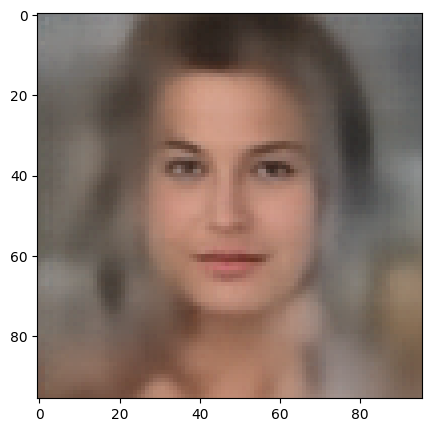# Autoencoders, latent space and the curse of high dimensionality – II – a view on fragments and filaments of the latent space for CelebA images

I continue with experiments regarding the structure which an Autoencoder [AE] builds in its latent space. In the last post of this series

Autoencoders, latent space and the curse of high dimensionality – I

we have trained an AE with images of the CelebA dataset. The Encoder and the Decoder of the AE consist of a series of convolutional layers. Such layers have the ability to extract characteristic patterns out of input (image) data and save related information in their so called feature maps. CelebA images show human heads against varying backgrounds. The AE was obviously able to learn the typical features of human faces, hair-styling, background etc. After a sufficient number of training epochs the AE’s Encoder produces “z-points” (vectors) in the latent space. The latent space is a vector space which has a relatively low number of dimension compared with the number of image pixels. The Decoder of the AE was able to reconstruct images from such z-points which resembled the original closely and with good quality.

We saw, however, that the latent space (or “z-space”) lacks an important property:

The latent space of an Autoencoder does not appear to be densely and uniformly populated by the z-points of the training data.

We saw that his makes the latent space of an Autoencoder almost unusable for creative and generative purposes. The z-points which gave us good reconstructions in the sense of recognizable human faces appeared to be arranged and positioned in a very special way within the latent space. Below I call a CelebA related z-point for which the Decoder produces a reconstruction image with a clearly visible face a “meaningful z-point“.

We could not reconstruct “meaningful” images from randomly chosen z-points in the latent space of an Autoencoder trained on CelebA data. Randomly in the sense of random positions. The Decoder could not re-construct images with recognizable human heads and faces from almost any randomly positioned z-point. We got the impression that many more non-meaningful z-points exist in latent space than meaningful z-points.

We would expect such a behavior if the z-points for our CelebA training samples were arranged in tiny fragments or thin (and curved) filaments inside the multidimensional latent space. Filaments could have the structure of

• multi-dimensional manifolds with almost no extensions in some dimensions
• or almost one-dimensional string-like manifolds.

The latter would basically be described by a (wiggled) thin curve in the latent space. Its extensions in other dimensions would be small.

It was therefore reasonable to assume that meaningful z-points are surrounded by areas from which no reasonable interpretable image with a clear human face can be (re-) constructed. Paths from a “meaningful” z-point would only in a very few distinct directions lead to another meaningful point. As it would be the case if you had to follow a path on a thin curved manifold in a multidimensional vector space.

So, we had some good reasons to speculate that meaningful data points in the latent space may be organized in a fragmented way or that they lie within thin and curved filaments. I gave my readers a link to a scientific study which supported this view. But without detailed data or some visual representations the experiments in my last post only provided indirect indications of such a complex z-point distribution. And if there were filaments we got no clue whether these were one- or multidimensional.

I have to correct this post regarding the basic line of thought: Even if we find that the z-points for CelebA images are arranged in filaments the failure we saw in the first post of this series may not have its direct cause in missing these filaments in latent space by randomly chosen z-points. It could also be that we miss a much larger, coherent region where meaningful points are located. The filaments then would correspond to a correlation of certain features, only, which may not be decisive for the reconstruction of a face. So, the investigation of the existence of filaments is interesting – but the explanation of the AE’s reconstruction failure may require a more thorough analysis. I have done the calculations already, but have not yet found the time to write about them. As soon as the posts are ready I am going to provide a link. See also an added comment at the end of this post.

Do we have a chance to get a more direct evidence about a fragmented or filamental population of the latent space? Yes, I think so. And this is the topic of this post.

However, the analysis is a bit complicated as we have to deal with a multidimensional space. In our case the number of dimensions of the latent space is z_dim = 256. No chance to plot any clusters or filaments directly! However, some other methods will help to reduce the dimensionality of the problem and still get some valid representations of the data point correlations. In the end we will have a very strong evidence for the existence of filaments in the AE’s z-space.

# Methods to work with data distributions in many dimensions

Below I will use several methods to investigate the z-point distribution in the multidimensional latent space:

• An analysis of the variation of the z-point number-density along coordinate axes and vs. radius values.
• An application of t-SNE projections from the standard multidimensional coordinate system onto a 2-dimensional plane.
• PCA analysis and subsequent t-SNE projections of the PCA-transformed z-point distribution and its most important PCA components down to a 2-dim plane. Note that such an approach corresponds to a sequence of projections:
1) Linear projections onto PCA rotated coordinates.
2) A non-linear SNE-projection which scales and represents data point correlations on different scales on a 2-dim plane.
• A direct view on the data distribution projected onto flat planes formed by two selected coordinate axes in the PCA-coordinate system. This will directly reveal whether the data (despite projection effects exhibit filaments and voids on some (small ?) scales.
• A direct view on the data distribution projected onto a flat plane formed by two coordinate axes of the original latent space.

The results of all methods combined strongly support the claim that the latent space is neither populated densely nor uniformly on (small) scales. Instead data points are distributed along certain filamental structures around voids.

# Layer structure of the Autoencoder

Below you find the layer structure of the AE’s Encoder. It got four Conv2D layers. The Decoder has a corresponding reverse structure consisting of Conv2DTranspose layers. The full AE model was constructed with Keras. It was trained on CelebA for 24 epochs with a small step size. The original CelebA images were reduced to a size of 96×96 pixels.

# Number density of z-points vs. coordinate values

Each z-point can be described by a vector, whose components are given by projections onto the 256 coordinate axes. We assume orthogonal axes. Let us first look at the variation of the z-point number density vs. reasonable values for each of the 256 vector-components.

Below I have plotted the number density of z-points vs. coordinate values along all 256 coordinate axes. Each curve shows the variation along one of the 256 axes. The data sampling was done on intervals with a width of 0.25: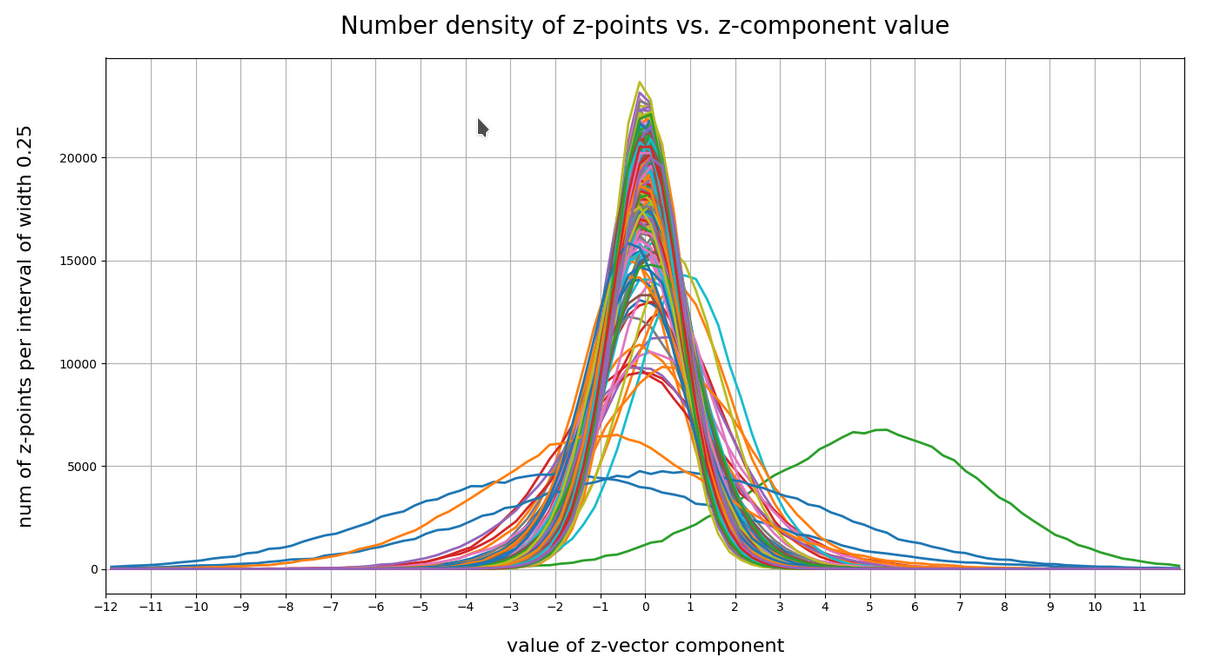Most curves look like typical Gaussians with a peak at the coordinate value 0.0 with a half-width of around 2.

You see, however, that there are some coordinates which dominate the spatial distribution in the latent vector-space. For the following components the number density distribution is relatively broad and peaks at a center different from the origin of the z-space. To pick a few of these coordinate axes:

``` 52, center:  5.0,  width: 8
61; center;  1.0,  width: 3
73; center:  0.0,  width: 5.5
83; center: -0.5,  width: 5
94; center:  0.0,  width: 4
116; center:  0.0,  width: 4
119; center:  1.0,  width: 3
130; center: -2.0,  width: 9
171; center:  0.7,  width: 5
188; center:  0.75, width: 2.75
200; center:  0.5,  width: 11
221; center: -1.0,  width: 8
```

The first number is just an index of the vector component and the related coordinate axis. The next plot shows the number density along some these specific coordinate axes:

What have we learned?
For most coordinate axes of the latent space the number density of the z-points peaks at 0.0. We see an approximate Gaussian form of the number density distribution. There are around 5 coordinate directions where the distribution has a peak significantly off the origin (52, 130, 171, 200, 221). Along the corresponding axes the distribution of z-points obviously has an elongated form.

If there were only one such special vector component then we would speak of an elongated, ellipsoidal and almost cigar like distribution with the thickest area at some position along the specific coordinate axis. For a combination of more axes with elongated distributions, each with with a center off the origin, we get instead diagonally oriented multidimensional and elongated shapes.

These findings show again that large regions of the latent space of an AE remain empty. To get an idea just imagine a three dimensional space with all data in x-direction culminating at a coordinate value of 5 with a half-width of lets say 8. In the other directions y and z we have our Gaussian distributions with a total half-width of 1 around the mean value 0. What do we get? A cigar like shape confined around the x-axis and stretching from -3 < x < 13. And the rest of the space: More or less empty. We have obviously found something similar at different angular directions of our multidimensional latent space. As the number of special coordinate directions is limited these findings tell us that a PCA analysis could be helpful. But let us first have a look at the variation of number density with the radius value of the z-points.

# Number density of z-points vs. radius

We define a radius via an Euclidean L2 norm for our 256-dimensional latent space. Afterward we can reduce the visualization of the z-point distribution to a one dimensional problem. We can just plot the variation of the number density of z-points vs. the radius of the z-points.

In the first plot below the sampling of data was done on intervals of 0.5 .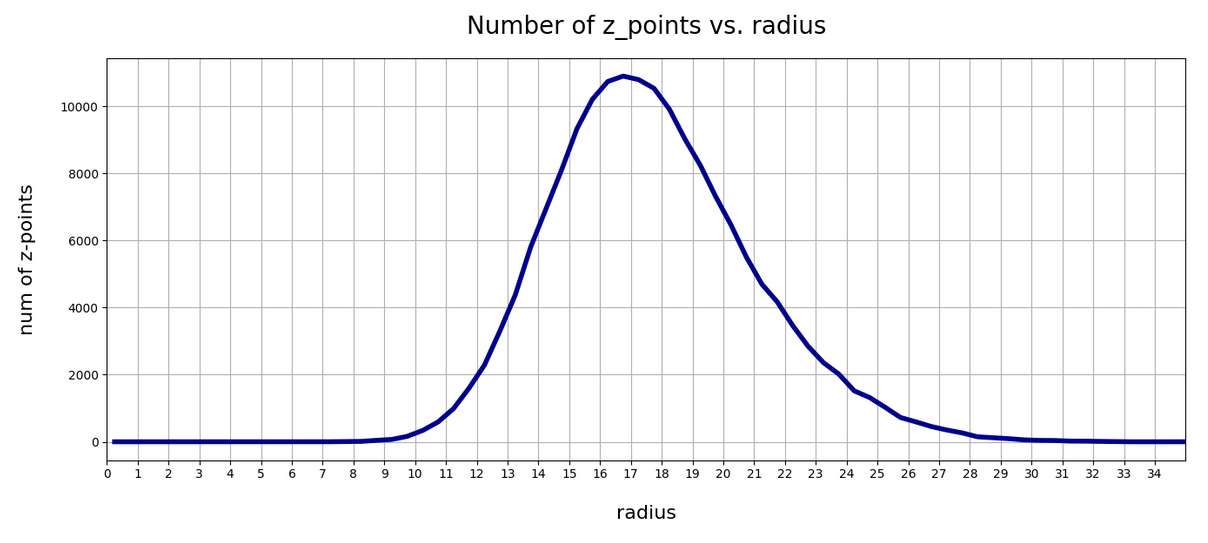The curve does not remain that smooth on smaller sampling intervals. See e.g. for intervals of width 0.05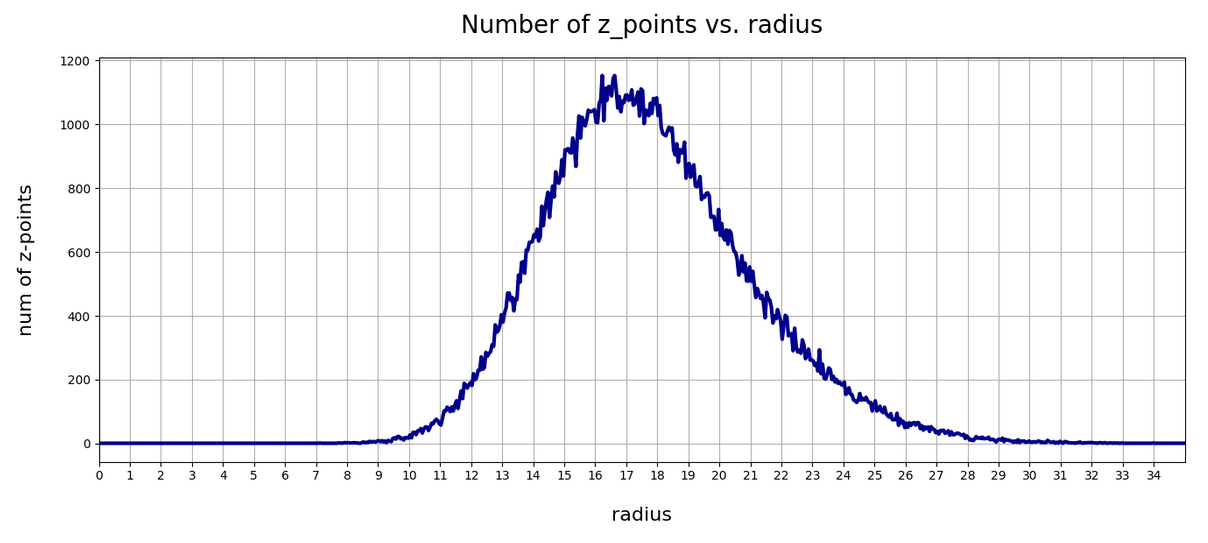Still, we find a pronounced peak at a radius of R=16.5. But do not get misguided: 16 appears to be a big value. But this is mainly due to the high number of dimensions!

How does the peak in the close vicinity of R=16 fit to the above number density data along the coordinate axes? Answer: Very well. If you assume a z-point vector with an average value of 1 per coordinate direction we actually get a radius of exactly R=16!

But what about Gaussian distributions along the coordinate axes? Then we have to look at resulting expectation values. Let us assume that we fill a vector of dimension 256 with numbers for each component picked statistically from a normal distribution with a width of 1. And let us repeat this process many times. Then what will the expectation value for each component be?

A coordinate value contributes with its square to the radius. The math, therefore, requires an evaluation of the integral integral[(x**2)*gaussian(x)] per coordinate. This integral gives us an expectation value for the contribution of each coordinate to the total vector length (on average). The integral indeed has a resulting value of 1.0. From this it follows that the expectation value for the distance according to an Euclidean L2-metric would be avg_radius = sqrt(256) = 16. Nice, isn’t it?

However, due to the fact that not all Gaussians along the coordinate axes peak at zero, we get, of course, some deviations and the flank of the number distribution on the side of larger radius values becomes relatively broad.

What do we learn from this? Regions very close to the origin of the z-space are not densely populated. And above a radius value of 32, we do not find z-points either.

# t-SNE correlation analysis and projections onto a 2-dimensional plane

To get an impression of possible clustering effects in the latent space let us apply a t-SNE analysis. A non-standard parameter set for the sklearn-variant of t-SNE was chosen for the first analysis

```tsne2 = TSNE(n_components, early_exaggeration=16, perplexity=10, n_iter=1000)
```

The first plot shows the result for 20,000 randomly selected z-points corresponding to CelebA images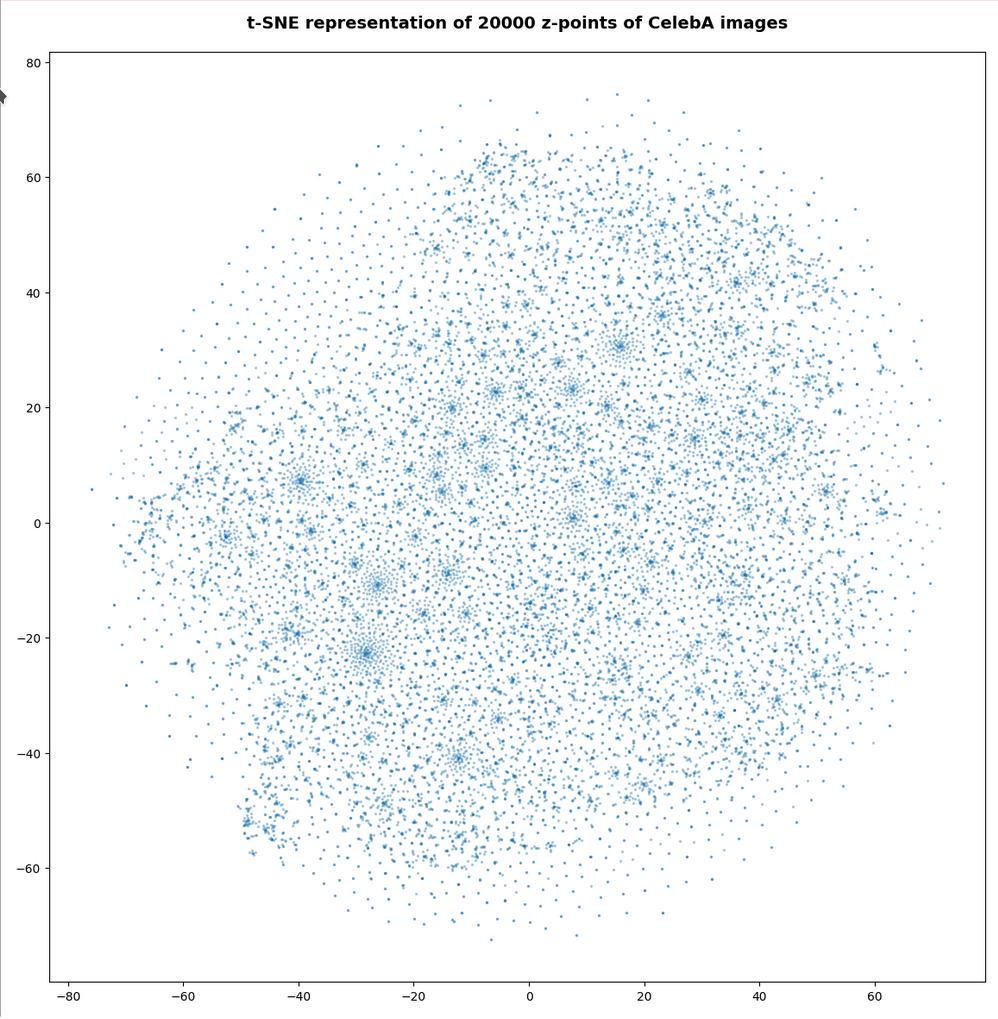Also this plot indicates that the latent space is not populated with uniform density in all regions. Instead we see some fragmentation and clustering. But note that this might happened on different length scales. t-SNE arranges its projections such that correlations on different scales get clearly indicated. So the distances in this plot must not be confused with the real spatial distances in the original latent space. The axes of the t-SNE plot do not reflect any axes of the latent space and the plotted distribution is not the real data point distribution after a linear and orthogonal projection onto a plane. t-SNE works non-linearly.

However, the impression of clustering remains for a growing numbers of z-points. In contrast to the first plot the next plots for 80,000 and 165,000 z-points were calculated with standard t-SNE parameters.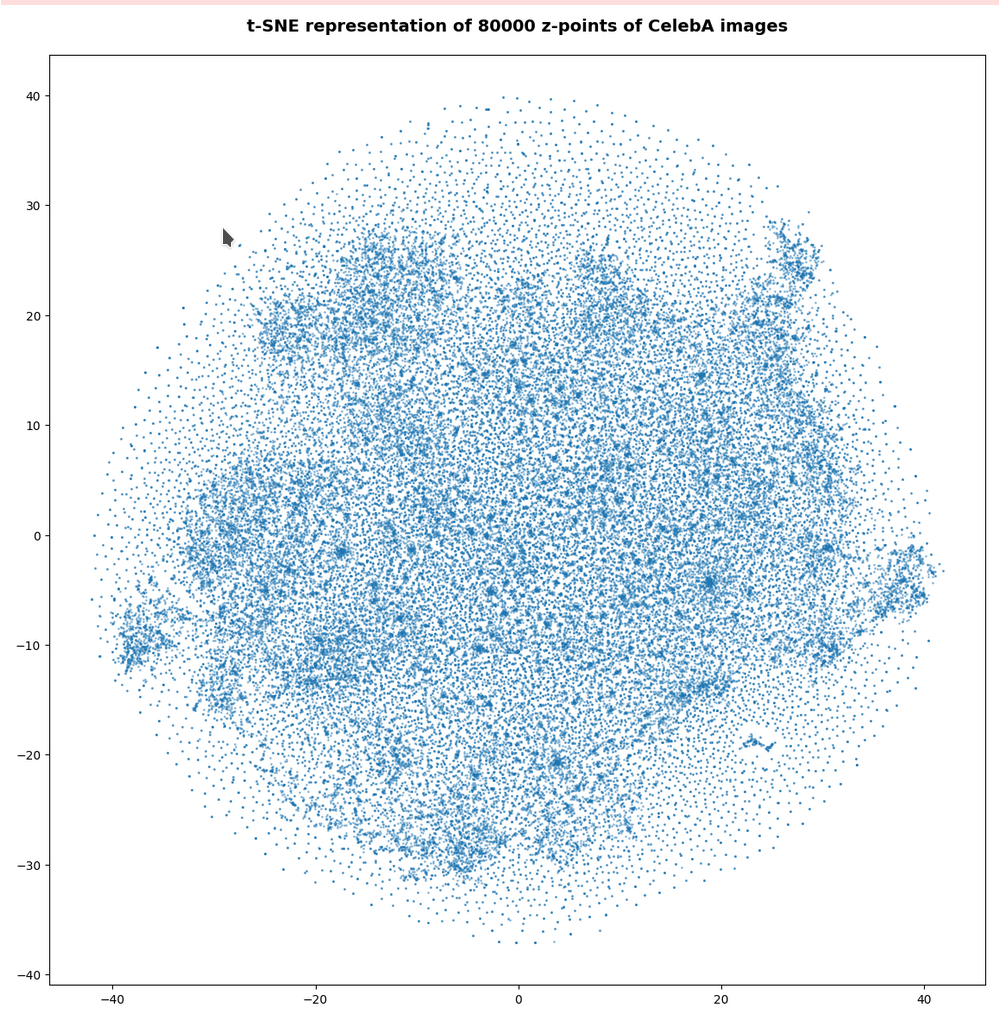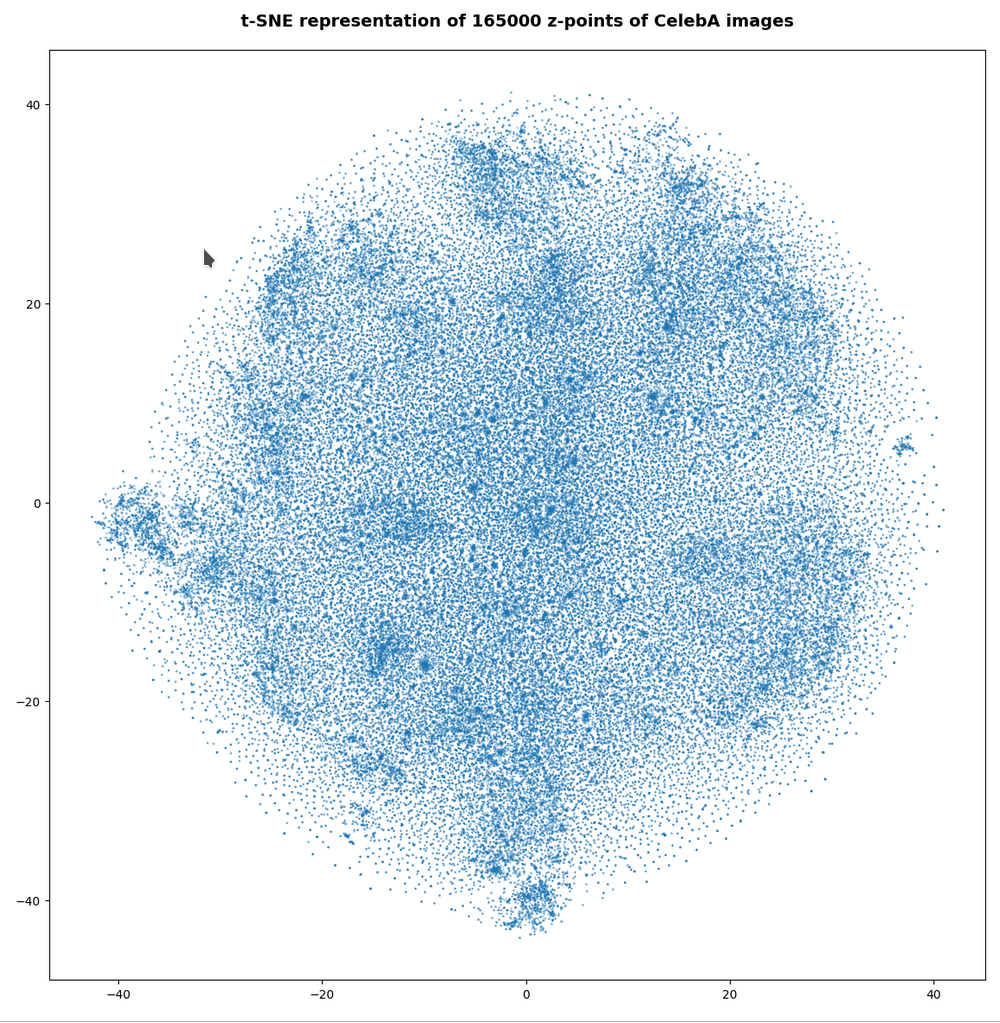We still see gaps everywhere between locally dense centers. At the center the size of the plotted points leads to overlapping. If one could zoom into some of the centers then gaps would again appear on smaller scales (see more plots below).

# PCA analysis and t-SNE-plots of the z-point distribution in the (rotated) PCA coordinate system

The z-point distribution can be analyzed by a PCA algorithm. There is one dominant component and the importance smooths out to an almost constant value after the first 10 components.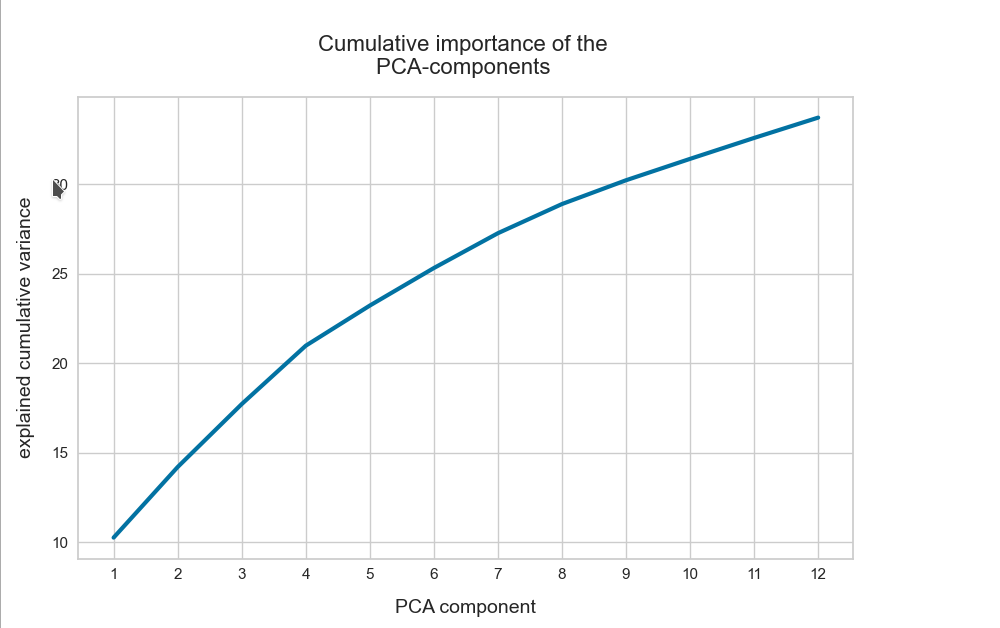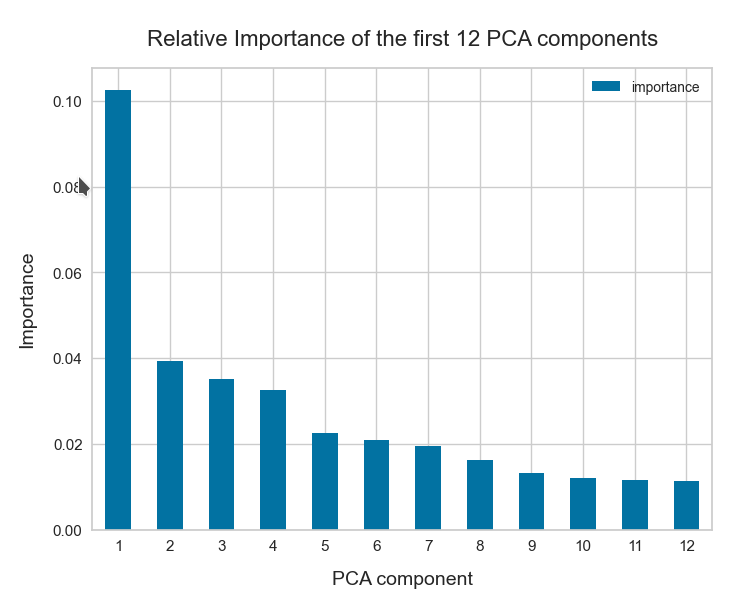This is consistent with the above findings. Most of the coordinates show rather similar Gaussian distributions and thus contribute in almost the same manner.

The PCA-analysis transforms our data to a rotated coordinate system with a its origin at a position such that the transformed z-point distribution gets centered around this new origin. The orthogonal axes of the new PCA-coordinates system show into the direction of the main components.

When the projection of all points onto planes formed by two selected PCA axes do not show a uniform distribution but a fragmented one, then we can safely assume that there really is some fragmentation going on.

## t-SNE after PCA

Below you see t-SNE-plots for a growing number of leading PCA components up to 4. The filamental structure gets a bit smeared out, but it does not really disappear. Especially the elongated empty regions (voids) remain clearly visible.

t-SNE after PCA for the first 2 main components – 80,000 randomly selected z-points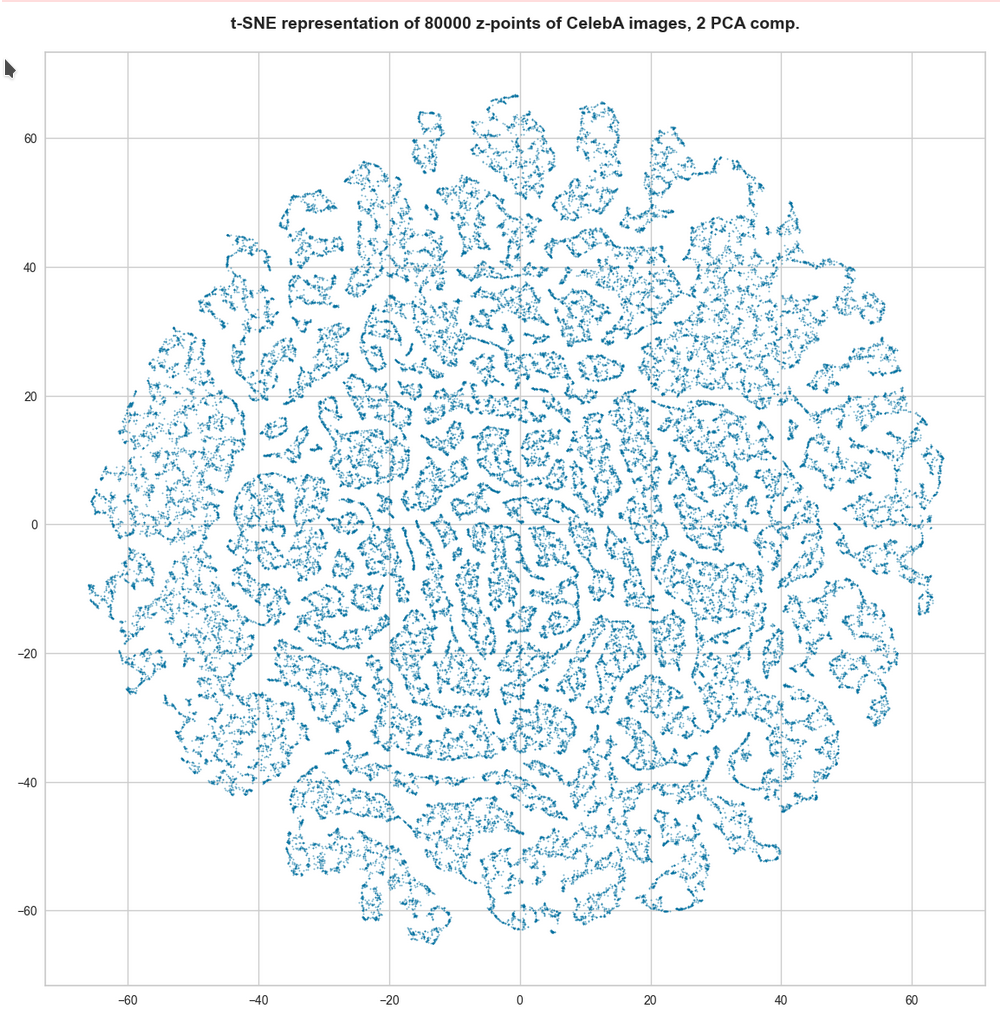t-SNE after PCA for the first 2 main components – 165,000 randomly selected z-points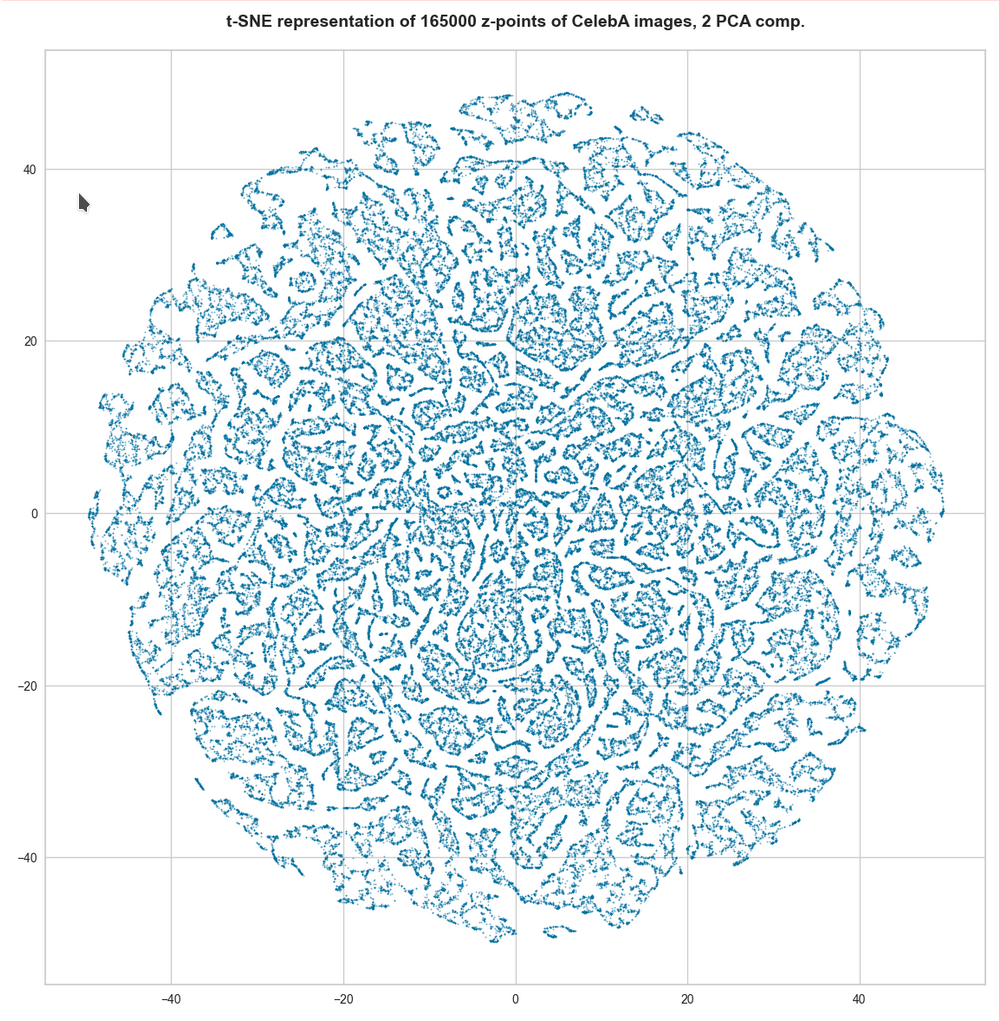t-SNE after PCA for the first 4 main PCA components – 165,000 randomly selected z-points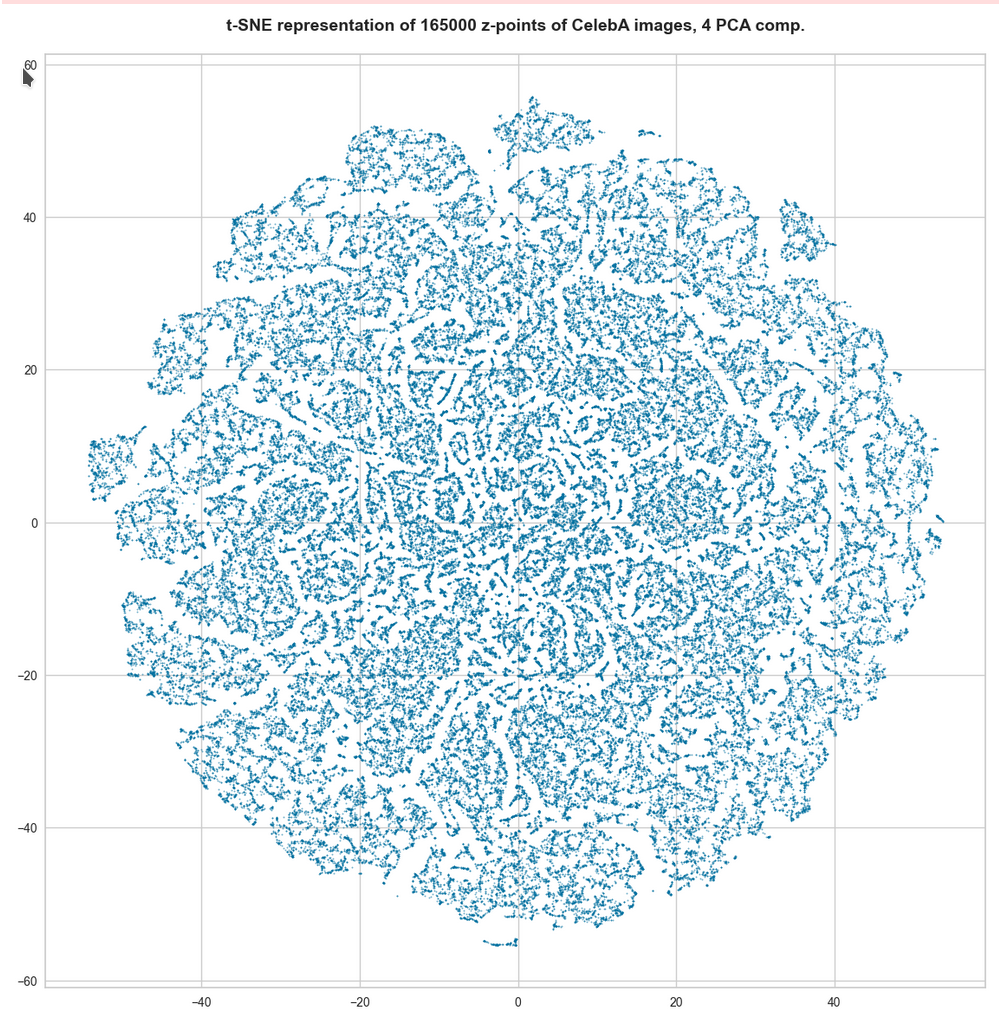For 10 components t-SNE gets a presentation problem and the plots get closer to what we saw when we directly operated on the latent space.But still the 10-dim space does not appear to be uniformly populated. Despite an expected smear out effect due to the non-linear projection the empty ares seem to be at least as many and as extended as the populated areas.

# Direct view on the z-point distribution after PCA in the rotated and centered PCA coordinate system

t-SNE blows correlations up to make them clearly visible. Therefore, we should also answer the following question:

On what scales does the fragmentation really happen ?

For this purpose we can make a scatter plot of the projection of the z-points onto a plane formed by the leading two primary component axes. Let us start with an overview and relatively large limiting values along the two (PCA) axes: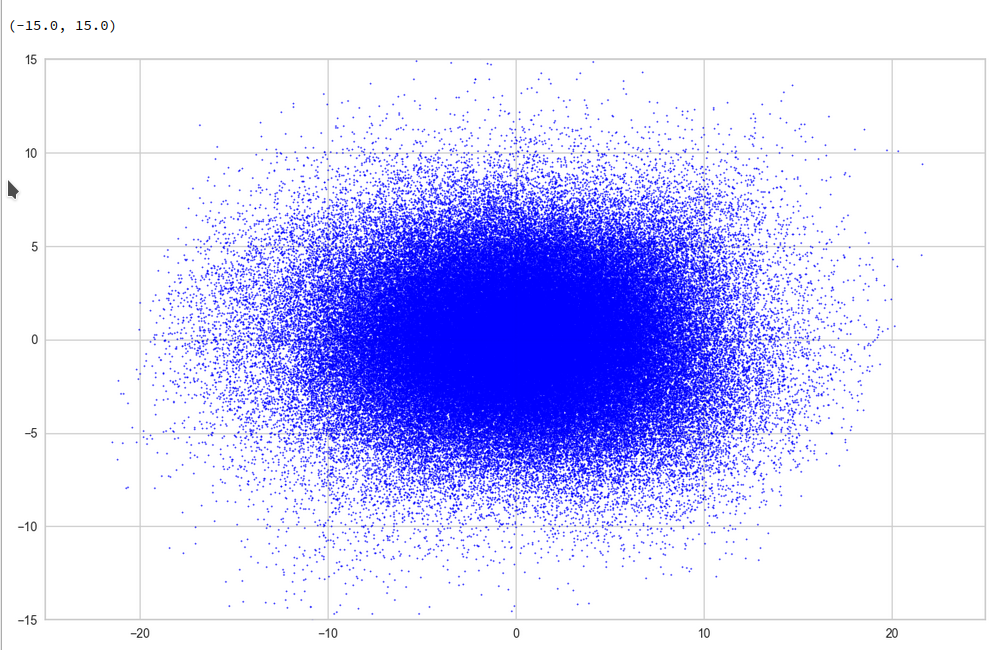Yeah, a PCA transformation obviously has centered the distribution. But now the latent space appears to be filled densely and uniformly around the new origin. Why?

Well, this is only a matter of the visualized length scales. Let us zoom in to a square of side-length 5 at the center: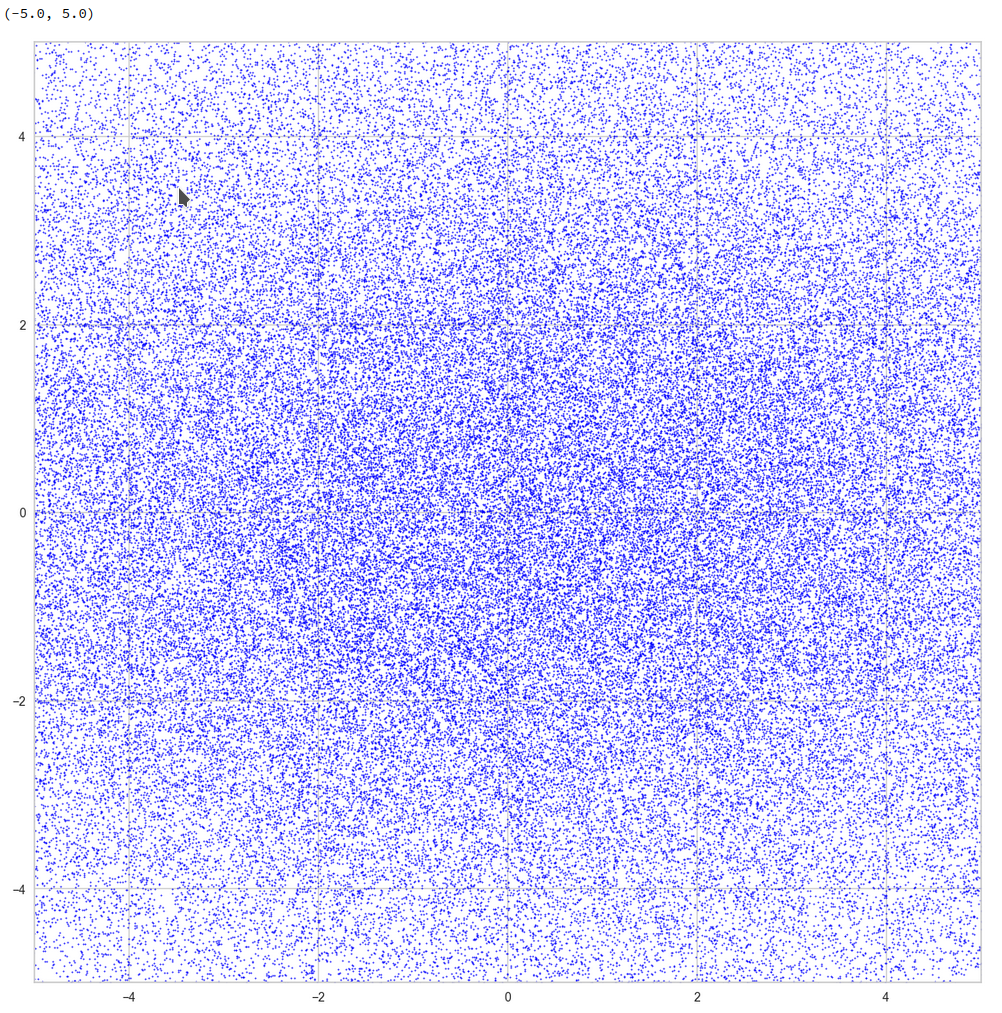Well, not so densely populated as we thought.

And yet a further zoom to smaller length scales: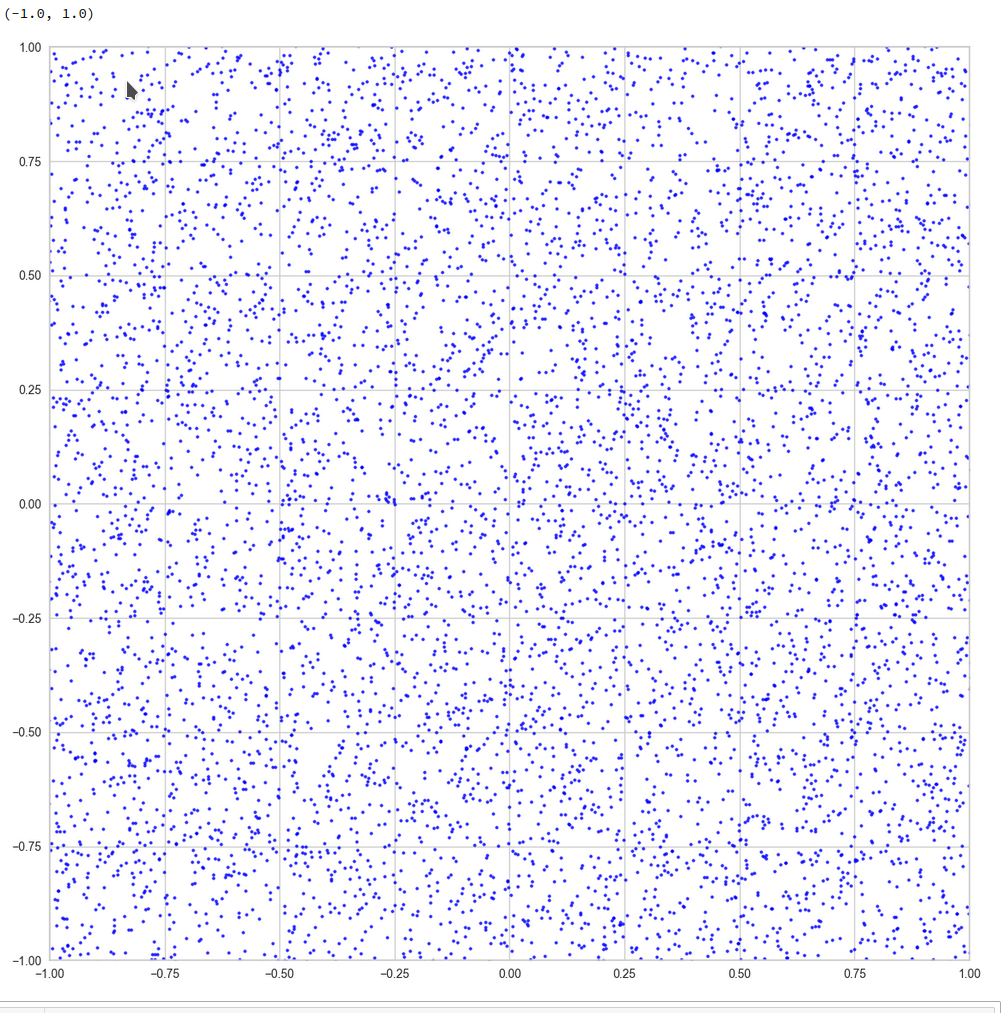And eventually a really small square around the origin of the PCA coordinate system:

z-point distribution at the center of a two-dim plane formed by the coordinate axes of the first 2 primary components
The chosen qsquare has its corners at (-0.25, -0.25), (-0.25, 0.25), (0.25, -0.25), (0.25, 0.25).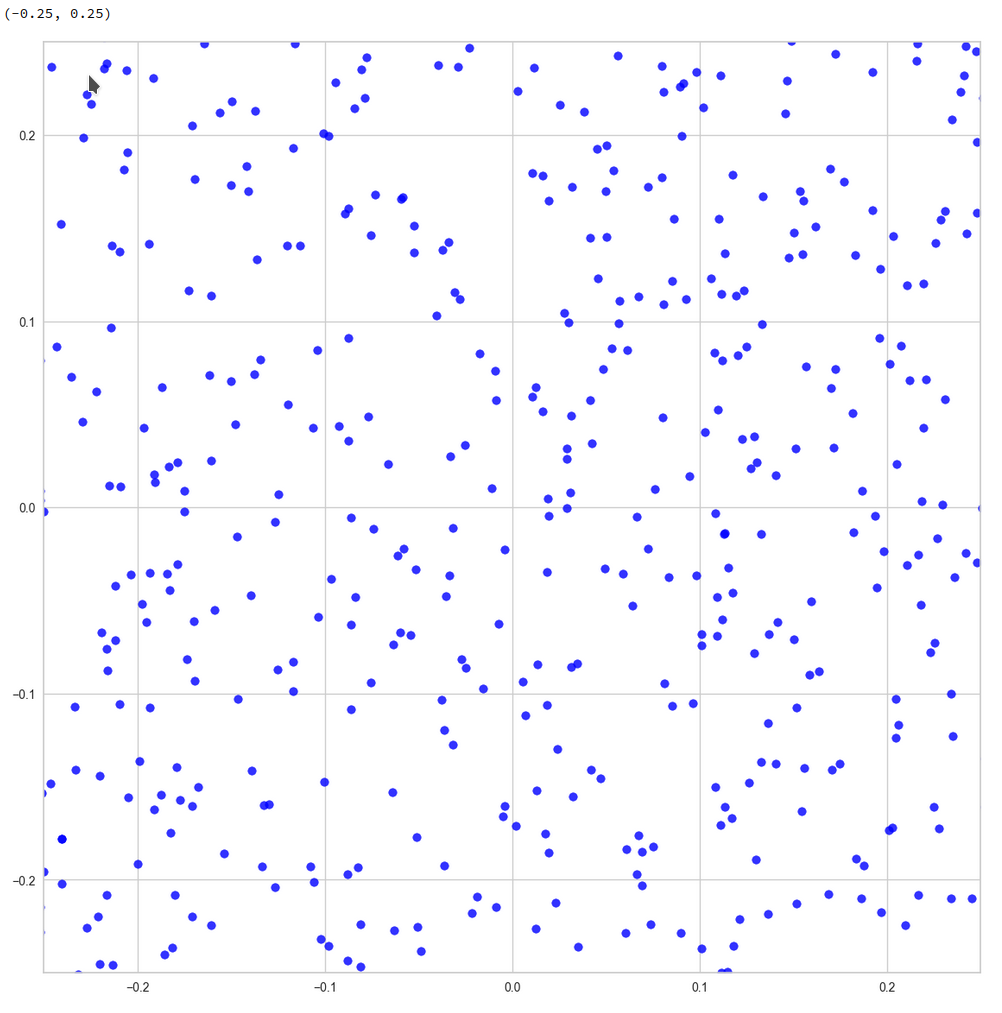Obviously, not a dense and neither a uniform distribution! After a PCA transformation we see the still see how thinly the latent space is populated and that the “meaningful” z-points from the CelebA data lie along curved and narrow lines or curves with some point-like intersections. Between such lines we see extended voids.

Let us see what happens when we look at the 2-dim pane defined by the first and the 18th axes of the PCA coordinate system: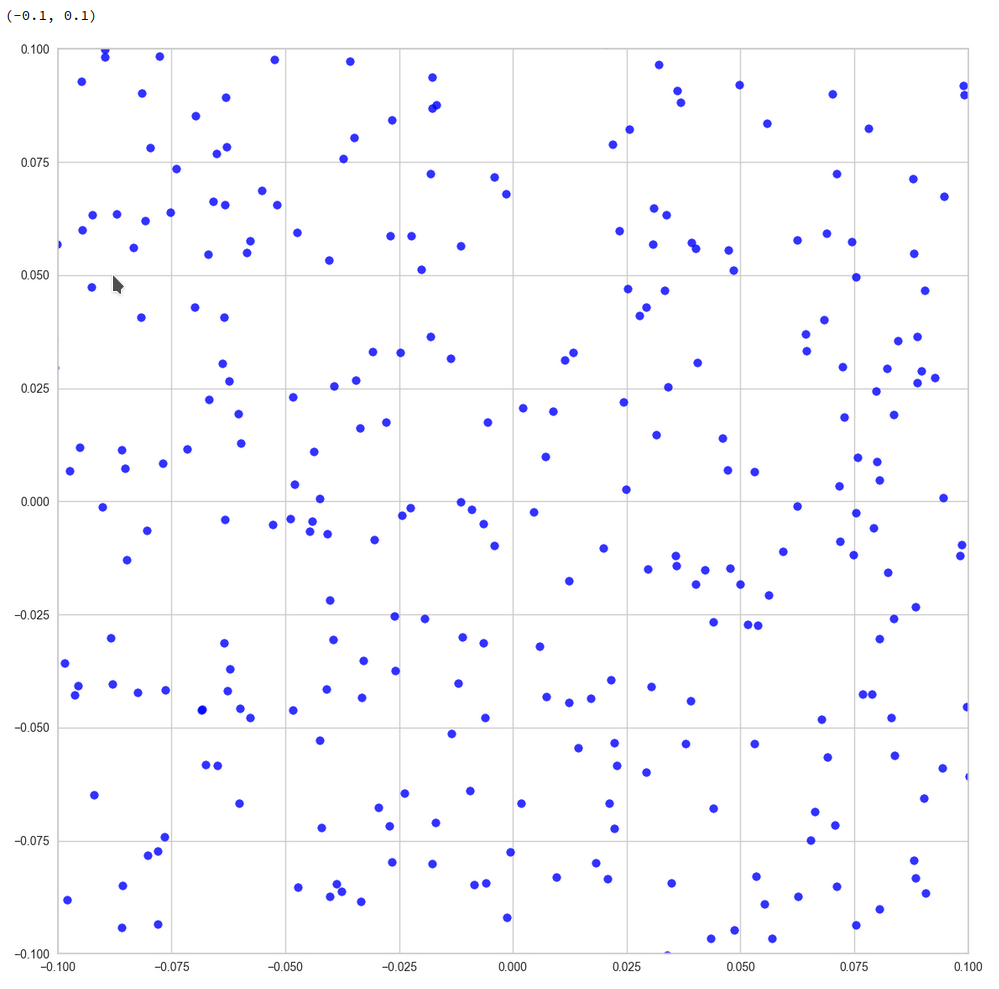Or the distribution resulting for the plane formed by the 8th and the 35th PCA axis: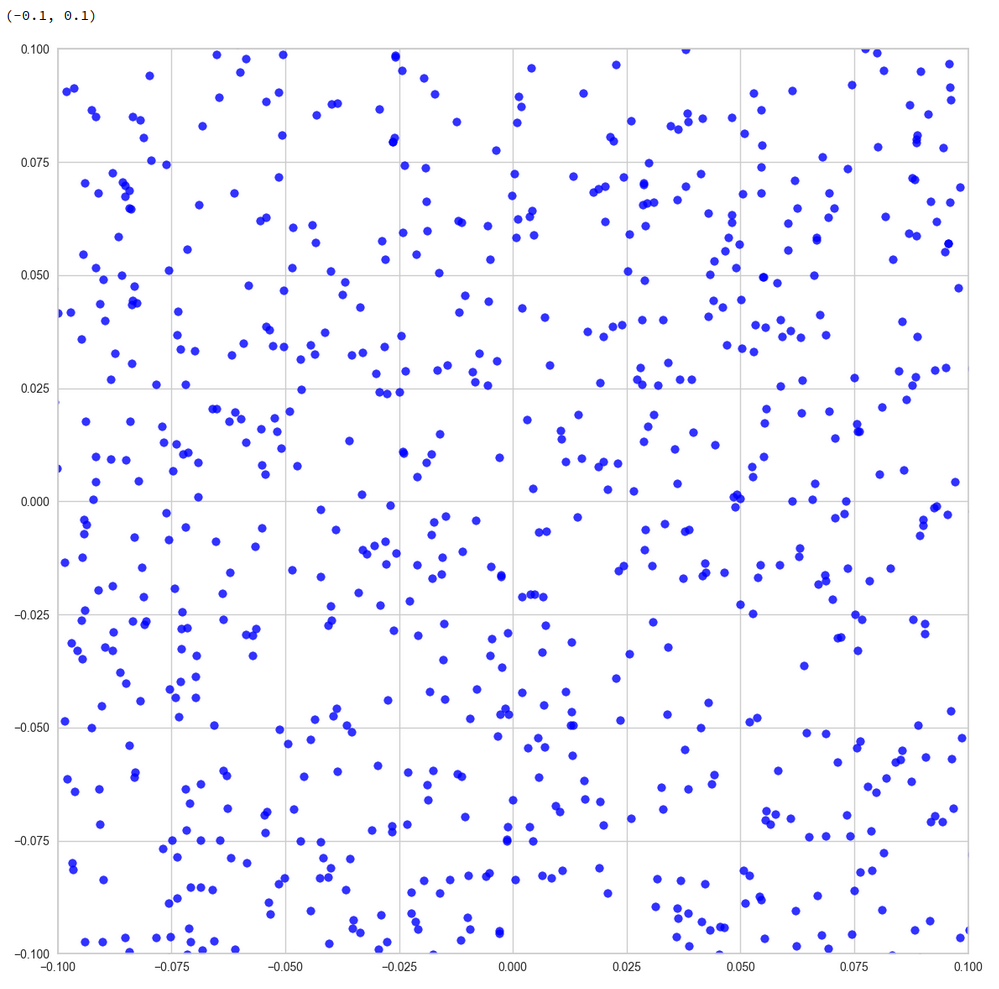We could look at other flat planes, but we do not get rid of he line like structures around void like areas. This is really a strong indication of filamental structures.

Interpretation of the line patterns:
The interesting thing is that we get lines for z-point projections onto multiple planes. What does this tell us about the structure of the filaments? In principle we have the two possibilities already named above: 1) Thin multidimensional manifolds or 2) thin and basically one-dimensional manifolds. If you think a bit about it, you will see that projections of multidimensional manifolds would not give us lines or curves on all projection planes. However curved string- or tube-like manifolds do appear as lines or line segments after a projection onto almost all flat planes. The prerequisite is that the extension of the string in other directions than its main one must really be small. The filament has to have a small diameter in all but one directions.

So, if the filaments really are one-dimensional string-like objects: Should we not see something similar in the original z-space? Let us for example look at the plane formed by axis 52 and axis 221 in the original z-space (without PCA transformation). You remember that these were axes where the distribution got elongated and had centers at -2 and 5, respectively. And indeed:

Again we see lines and voids. And this strengthens our idea about filaments as more or less one-dimensional manifolds.

The “meaningful” z-points for our CelebA data obviously get positioned on long, very thin and basically one-dimensional filaments which surround voids. And the voids are relatively large regarding their area/volume. (Reminds me of the galaxy distribution in simulations of the development of the early universe, by the way.)

Therefore: Whenever you chose a randomly positioned z-point the chance that you end up in an unpopulated region of the z-space or in a void and not on a filament is extremely big.

# Conclusion

We have used a whole set of methods to analyze the z-point distribution of an AE trained on CelebA images. We found the the z-point distribution is dominated by the number density variation along a few coordinate axes. Elongated shapes in certain directions of the latent space are very plausible on larger scales.

We found that the number density distributions along most of the coordinate axes have a thin Gaussian form with a peak at the origin and a half-with of 1. We have no real explanation for this finding. But it may be related to the fact the some dominant features of human faces show Gaussian distributions around a mean value. With Gaussians given we could however explain why the number density vs. radius showed a peak close to R=16.

A PCA analysis finds primary directions in the multidimensional space and transforms the z-point distribution into a corresponding one for orthogonal primary components axes. For logical reason we can safely assume that the corresponding projections of the z-point distribution on the new axes would still reveal existing thin filamental structures. Actually, we found lines surrounding voids independently onto which flat plane we projected the data. This finding indicates thin, elongated and curved but basically one-dimensional filaments (like curved strings or tubes). We could see the same pattern of line-like structure in projections onto flat coordinate planes in the original latent space. The volume of the void areas is obviously much bigger than the volume occupied by the filaments.

Non-linear t-SNE projections onto a 2-dim flat hyperplanes which in addition reproduce and normalize correlations on multiple scales should make things a bit fuzzier, but still show empty regions between denser areas. Our t-SNE projections all showed signs of complex correlation patterns of the z-points with a lot of empty space between curved structures.

The following original conclusion is misleading and by parts wrong:

The experiments all in all indicate that z-points of the training data, for which we get good reconstructions, lie within thin filaments on characteristic small length scales. The areas/volumes of the voids between the filaments instead are relatively big. This explains why chances that randomly chosen points in the z-space falls into a void are very high.
The results of the last post are consistent with the interpretation that z-points in the voids do not lead to reconstructions by the Decoder which exhibit standard objects of the training images. in the case of CelebA such z-points do not produce images with clear face or head like patterns. Face like features obviously correspond to very special correlations of z-point coordinates in the latent space. These correlations correspond to thin manifolds consuming only a tiny fraction of the z-space with a volume close zero.

Due to a new analysis I would like to replace my original statemets with a question:

Do our findings of the existence of filaments and large surrounding voids really explain the results of the first post that randomly chosen z-points miss areas in the latent space which allow for a reconstruction of “faces”?

I am going to answer this question in another better prepared post series, soon. To make you a bit curious I leave you with the fact that the following picture shows a face reconstructed by an AE from a randomly selected point in the latent space – with some simple conditions applied: